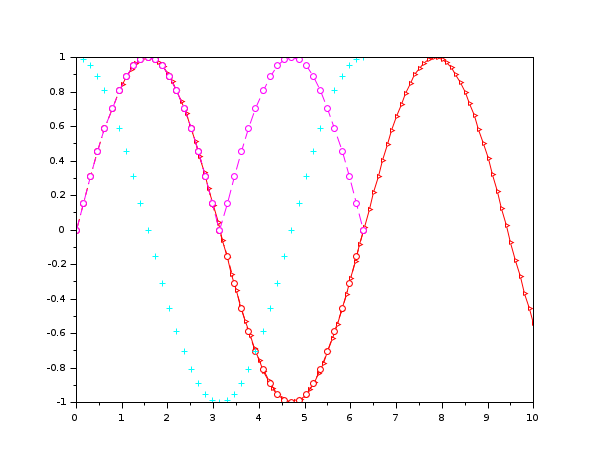Change language to:
English - 日本語 - Português - Русский

See the recommended documentation of this function

Aide de Scilab >> Graphiques > 2d_plot > LineSpec

# LineSpec

to quickly customize the lines appearance in a plot

### Description

The `LineSpec` is an optional argument that can be used inside a `plot` command to customize each new line aspect. It has to be given as a concatenated string containing information about color, line style or markers. It is very useful to quickly specify such basic line properties.

To specify a red longdash-dot with diamond marker, the string can be `'r-.diam'`. As you can see, a full complete spelling of each property value is not required but the string, which is a concatenation (in any order) of these three types of properties, must remain unambiguous. Furthermore, the string specification is not case sensitive.

Here is a complete list of the `LineSpec` types you can specify (using plot).

LineStyle:

a string defining the line style. This property is linked to the object's `line_style` property (see polyline properties).

 `Specifier` `Line Style` `-` Solid line (default) `--` Dashed line `:` Dotted line `-.` Dash-dotted line
Color:

a string defining the line color. This property is linked to the object's `foreground` property (see polyline properties).

 `Specifier` `Color` `r` Red `g` Green `b` Blue `c` Cyan `m` Magenta `y` Yellow `k` Black `w` White

A default color table is used to color plotted curves if you do not specify a color (neither with `LineSpec` nor with GlobalProperty). When drawing multiple lines, the plot command automatically cycles through this table. Here are the used colors:

 `R` `G` `B` 0. 0. 1. 0. 0.5 0. 1. 0. 0. 0. 0.75 0.75 0.75 0. 0.75 0.75 0.75 0. 0.25 0.25 0.25
Marker type:

A string defining the marker type. Note that if you specify a marker without a line style, only the marker is drawn. This property is linked to the object's `mark_style` and `mark_mode` properties (see polyline properties).

 `Specifier` `Marker Type` `+` Plus sign `o` Circle `*` Asterisk `.` Point `x` Cross `'square'` or `'s'` Square `'diamond'` or `'d'` Diamond `^` Upward-pointing triangle `v` Downward-pointing triangle `>` Right-pointing triangle `<` Left-pointing triangle `'pentagram' or 'p'` Five-pointed star (pentagram) No marker (default)

### Examples

```clf();
x=1:0.1:10; // Init.
plot(x,sin(x),'r-.>') // plots a dash-dotted line with a right-pointing triangle centered on each points.``````clf();
x=1:0.1:10;
// If you specify a marker without a line style, only the marker is drawn
plot(x,sin(x),'d')``````clf();
x = 1:0.5:10; // Init.
// The order of information about color, line style or markers does not matter
// BUT the information must be unambiguous
subplot(311);plot(x,sin(x),'.b-');
subplot(312);plot(x,sin(x),'b.-');
subplot(313);plot(x,sin(x),'b-.');// The point belongs to information about line style (not marker!)``````//multiple plots with different LineSpecs
clf();
t=0:%pi/20:2*%pi;
plot(t,sin(t),'ro-.',t,cos(t),'cya+',t,abs(sin(t)),'--mo')```